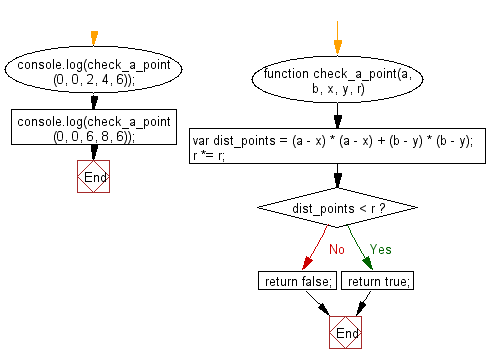# JavaScript: Check whether a point lies strictly inside a given circle

## JavaScript Basic: Exercise-120 with Solution

Write a JavaScript program to check whether a point lies strictly inside a given circle.

Input:
Center of the circle (x, y)
Point inside a circle (a, b)

Sample Solution:

HTML Code:

``````<!DOCTYPE html>
<html>
<meta charset="utf-8">
<meta name="viewport" content="width=device-width">
<title> Check whether a point lies strictly inside a given circle</title>
<body>

</body>
</html>
```
```

JavaScript Code:

``````function check_a_point(a, b, x, y, r) {
var dist_points = (a - x) * (a - x) + (b - y) * (b - y);
r *= r;
if (dist_points < r) {
return true;
}
return false;
}

console.log(check_a_point(0, 0, 2, 4, 6));
console.log(check_a_point(0, 0, 6, 8, 6));
``````

Sample Output:

```true
false
```

Flowchart:ES6 Version:

``````function check_a_point(a, b, x, y, r) {
var dist_points = (a - x) * (a - x) + (b - y) * (b - y);
r *= r;

if (dist_points < r) {
return true;
}

return false;
}

console.log(check_a_point(0, 0, 2, 4, 6));
console.log(check_a_point(0, 0, 6, 8, 6));
``````

Live Demo:

See the Pen javascript-basic-exercise-120 by w3resource (@w3resource) on CodePen.

Improve this sample solution and post your code through Disqus

What is the difficulty level of this exercise?

Test your Programming skills with w3resource's quiz.

﻿

## JavaScript: Tips of the Day

Checks if a string is an anagram of another string (case-insensitive, ignores spaces, punctuation and special characters)

Example:

```const isAnagram = (str1, str2) => {
const normalize = str =>
str
.toLowerCase()
.replace(/[^a-z0-9]/gi, '')
.split('')
.sort()
.join('');
return normalize(str1) === normalize(str2);
};
console.log(isAnagram('iceman', 'cinema')); // true
```

Output:

```true
```